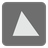Similar Triangles
Moderate
English

This is a mission to check the similarity of two triangles.

You are given two lists as coordinates of vertices of each triangle.
You have to return a bool. (The triangles are similar or not)

Example:

```similar_triangles([(0, 0), (1, 2), (2, 0)], [(3, 0), (4, 2), (5, 0)]) is True
similar_triangles([(0, 0), (1, 2), (2, 0)], [(3, 0), (4, 3), (5, 0)]) is False
similar_triangles([(1, 0), (1, 2), (2, 0)], [(3, 0), (5, 4), (5, 0)]) is True
```Input:

• Two lists as coordinates of vertices of each triangle.
• Coordinates is three tuples of two integers.

Output: True or False.

Precondition:

• -10 ≤ x(, y) coordinate ≤ 10